# Chapter 8.2. Exam Preparation - Part II

In the current chapter, we will review a practical exam in Programming Basics, conducted at SoftUni on December 18, 2016. The problems will give you a good overview of what you can expect at an admission exam in Programming at SoftUni. The exam covers the material studied in the current book.

## Exam Problems

Traditionally, the admission exam at SoftUni consists of 6 practical programming problems:

• Simple problems (no conditions).
• A problem with a single condition.
• A problem with more complex conditions.
• A problem with a single loop.
• A problem with nested loops (drawing a figure on the console).
• A problem with nested loops and more complex logic.

Let's examine a real exam topic, the problems it contains, and their solutions.

## Problem: Distance

Write a program that calculates what is the distance passed by a car (in kilometers), if we know the initial speed (km/h), the initial time frame in minutes, then the speed is increased by 10%, the second time frame, then the speed is decreased by 5%, and the time until the end of the trip. To calculate the distance, you need to convert the minutes into hours (for example 70 minutes = 1.1666 hours).

### Input Data

Read 4 lines from the console:

• The start speed in km/h – an integer within the range [1 … 300].
• The first time in minutes – an integer within the range [1 … 1000].
• The second time in minutes – an integer within the range [1 … 1000].
• The third time in minutes – an integer within the range [1 … 1000].

### Output Data

Print a number on the console: the kilometers passed, formatted up to the second digit after the decimal point.

### Sample Input and Output

90
60
70
80
330.90 Distance with initial speed: 90 km/h * 1 hour (60 min) = 90 km
After speed increase: 90 + 10% = 99.00 km/h * 1.166 hours (70 min) = 115.50 km
After speed decrease: 99 - 5% = 94.05 km/h * 1.33 hours (80 min) = 125.40 km
Total number of km passed: 330.9 km
140
112
75
190
917.12 Distance with initial speed: 140 km/h * 1.86 hours (112 min) = 261.33 km
After speed increase: 140 + 10% = 154.00 km/h * 1.25 hours (75 min) = 192.5 km
After speed decrease: 154.00 - 5% = 146.29 km/h * 3.16 hours (190 min) = 463.28 km
Total number of km passed: 917.1166 km

### Hints and Guidelines

Such a description may look misleading and incomplete at first glance, which adds to the complexity of a relatively easy task. Let's separate the problem into a few sub-problems and try to solve each of them one by one, which will lead us to the final result:

• Receiving the input data.
• Execution of the main programming logic.
• Calculation and shaping up the final result.

The main part of the programming logic is to calculate what will be the distance passed after all speed changes. As during execution of the program, part of the data that we have is modified, we could separate the program code into a few logically separated parts:

• Calculation of the distance passed with initial speed.
• Change of speed and calculation of the distance passed.
• Last change of speed and calculation.
• Summing up.

On condition for input will be submitted four separate rows, for this reason, we will have to call the function input() four times: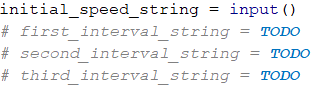Next, we have to convert the input data to a suitable type so we can do the needed computations. We choose type int because in the description of the problem is mentioned that the input data is going to be in a determined interval, for which this data type is enough. We perform the data conversion as follows: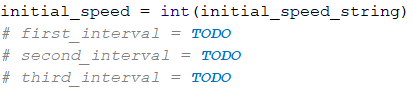In this way, we solved successfully the first sub-problem - receiving the input data.

We initially store one variable that will be used multiple times. This centralization approach gives us flexibility and the possibility to modify the final result of the program with minimum effort. In case we need to change the value, we must do it in only one place in the code, which saves us time and effort: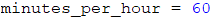Avoiding repetitive code (centralization of the program logic) in the tasks that we examine in the present book may look unnecessary at first glance, but this approach is very important upon building large applications in a real work environment, and its exercising in an initial stage of training will help you build a quality programming style.

We calculate the travel time (in hours) by dividing the time by 60 (minutes in an hour). The travel distance is calculated by multiplying the starting speed by the time passed (in hours). After that, we change the speed by increasing it by 10%(on condition), as per the task description. Calculating the percentage, as well as the following distances passed, is done in the following way:

• The time frame (in hours) is calculated by dividing the provided time frame in minutes by the minutes that are contained in an hour (60).
• The distance passed is calculated by multiplying the time frame (in hours) by the speed that is obtained after the increase.
• The next step is to decrease the speed by 5%, as per the problem description.
• We calculate the remaining distance in the manner described in the first two points.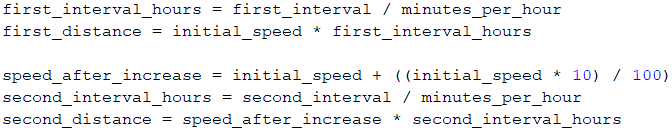Up until now, we were able to solve two of the most important sub-problems, namely the receiving data input and their processing. What remains is to calculate the final result. As by the description, we are required to format it up to 2 symbols after the decimal point, we can do this in the following manner: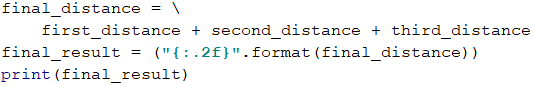In case you worked accurately and wrote the program using the input data given in the task description, you will be convinced that it works properly.

## Problem: Change Tiles

Haralambi has some savings that he wants to use to change the tiles on the bathroom floor. The floor is rectangular, and the tiles are triangular. Write a program that calculates if his savings will be sufficient. The width and length of the floor are submitted, as well as one of the sides of the triangle with its height towards it. We must calculate how many tiles are needed, to cover the floor. The number of tiles must be rounded up to the higher integer and 5 more tiles must be added as spare tiles. Also, we have submittedthe price per tile and the amount paid for the work of a workman.

### Input Data

Read 7 lines from the console:

• The savings.
• The width of the floor.
• The length of the floor.
• The side of the triangle.
• The height of the triangle.
• The price of a tile.
• The sum for the workman.

All numbers are real numbers within the range [0.00 … 5000.00].

### Output Data

In this problem, our currency will be lv, which is BGN (Bulgarian lev). The following must be printed on the console as a single line:

• If the money is sufficient:
• “{Remaining funds} left.”
• If the money IS NOT sufficient:
• “You'll need {Insufficient funds} lv more.”

The result must be formatted up to the second symbol after the decimal point.

### Sample Input and Output

500
3
2.5
0.5
0.7
7.80
100
25.60 lv left. Floor area → 3 * 2.5 = 7.5
Tile area → 0.5 * 0.7 / 2 = 0.175
Needed tiles → 7.5 / 0.175 = 42.857… = 43 + 5 spare tiles = 48
Total amount → 48 * 7.8 + 100 (workman) = 474.4
474.4 < 50025.60 lv (BGN) left
1000
5.55
8.95
0.90
0.85
13.99
321
You'll need 1209.65 lv more. Floor area → 5.55 * 8.95 = 49.67249
Tile area → 0.9 * 0.85 / 2 = 0.3825
Needed tiles → 49.67249 / 0.3825 = 129.86… = 130 + 5 spare tiles = 135
Total amount → 135 * 13.99 + 321 (workman) = 2209.65
2209.65 > 10001209.65 lv (BGN) are insufficient

### Hints and Guidelines

The following task requires our problem to accept more input data and to perform a larger amount of calculations, even though the solution is identical. Accepting the input data is done familiarly. Pay attention that in the input part of the description is said that all input data will be real numbers and for this reason, we will use float type.

Now that we already have everything for executing the programming logic, we can move to the following part. How can we calculate what is the needed number of tiles that will be sufficient to cover the entire floor? The requirement is that tiles have a triangular shape, which can confuse, but practically, the task needs just simple calculations. We can calculate the common part of the floor by the formula for finding a rectangle area, as well as the area of a single tile using the relevant formula for the triangle area.

To calculate the number of tiles that are needed, we divide the floor area by the area of a single tile (we should not forget to add the 5 additional tiles, that were mentioned in the requirements).Pay attention that the requirements state that we should round up the number of tiles, obtained upon the division, up to the higher number, and then we should add 5. Find more information about the system functionality that does that: math.ceil(…).

We can find the final result by calculating the total amount that is needed to cover the entire floor, by summing up the tile price and the price that will be paid to the workman, that we have from the input data. We can figure out that the total costs for tiles can be calculated by multiplying the number of tiles by the price per tile. We will find out whether the amount that we have will be sufficient by comparing the savings (based on the input data) and the total costs: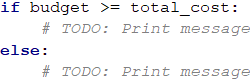## Problem: Flowers

A flowers shop offers 3 types of flowers: chrysanthemums, roses, and tulips. The prices depend on the season. In this problem, our currency will be lv, which is BGN (Bulgarian lev).

Season Chrysanthemums Roses Tulips
spring / summer
autumn / winter
2.00 lv./pc.
3.75 lv./pc.
4.10 lv./pc.
4.50 lv./pc.
2.50 lv./pc.
4.15 lv./pc.

On holidays, the prices of all flowers are increased by 15%. The following discounts are offered:

• For purchasing more than 7 tulips in spring – 5% of the price of the whole bouquet.
• For purchasing 10 or more roses in winter – 10% of the price of the whole bouquet.
• For purchasing more than 20 flowers in total in any season – 20% of the price of the whole bouquet.

Discounts are made in the above-described order and can be combined! All discounts are valid after increasing the price on a holiday!

The price for arranging a bouquet is always 2 lv (BGN). Write a program that calculates the price of a bouquet.

### Input Data

The input is read from the console and contains exactly 5 arguments:

• The number of the purchased chrysanthemums – integer number inside the interval of [0 … 200].
• The number of the purchased roses – integer number inside the interval of [0 … 200].
• The number of the purchased tulips – integer number inside the interval of [0 … 200].
• The season – [Spring, Summer, Autumn, Winter].
• If the day is a holiday – [Y - yes / N - no].

### Output Data

Print on the console 1 number – the price of flowers, formatted up to the second digit after the decimal point.

### Sample Input and Output

2
4
8
Spring
Y
46.14 Price: 2*2.00 + 4*4.10 + 8*2.50 = 40.40 BGN.
Holiday: 40.40 + 15% = 46.46 BGN.
5% discount for more than 7 tulips in spring: 44.14
The flowers are in total 20 or less: no discount
44.14 + 2 for arranging = 46.14 BGN.
3
10
9
Winter
N
69.39 Price: 3*3.75 + 10*4.50 + 9*4.15 = 93.60 BGN.
No holiday: no increase in price
10% discount for 10 or more roses in winter: 84.24
The flowers are in total over 20: 20% discount = 67.392
67.392 + 2 for arranging = 69.392 BGN.
Input Output
10
10
10
Autumn
N
101.20

### Hints and Guidelines

After carefully reading the requirements, we understand that once again we need to do simple calculations, however, this time we will need additional logical conditions. We need to pay more attention to the moment of making changes in the final price, to be able to properly build the logic of our program. Again, the bold text gives us sufficient guidelines on how to proceed. For a start, we will separate the already defined values in variables, as we did in the previous tasks: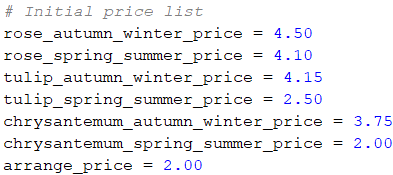We will also do the same for the rest of the defined values: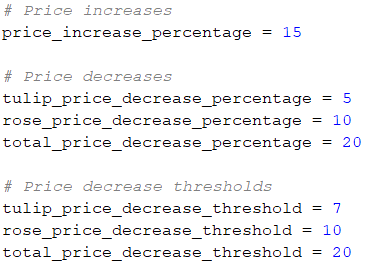Our next sub-task is to read properly the input data from the console. We will do that in an already familiar manner but this way we combine two separate functions - one to read a line from the console and another to convert in a numeric data type: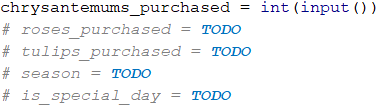Let's think of the most appropriate way to structure our programming logic. By the requirements, it becomes clear that the path of the program is divided mainly into two parts: spring / summer and autumn / winter. We can do the separation by a conditional statement, by storing variables in advance for the prices of the individual flowers, as well as for the final result: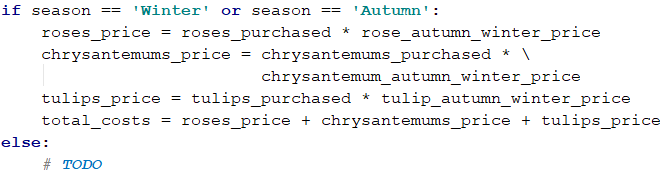What remains is to perform a few checks regarding the discounts of the different types of flowers, depending on the season, and to modify the final result.

### Testing in The Judge System

Write a program that calculates statistics for grades in an exam. In the beginning, the program receives the number of students who attended the exam and for each student – their grade. In the end, the program must print the percentage of students that have grades between 2.00 and 2.99, between 3.00 and 3.99, between 4.00 and 4.99, 5.00 or more, as well as the average grade of the exam.

### Input Data

On the console are being read a sequence of numbers, each on a different row:

• On the first line – the number of students who attended the exam – an integer within the range [1 … 1000].
• For each student on a separate line – the grade on the exam – a real number within the range [2.00 … 6.00].

### Output Data

Print on the console 5 lines that hold the following information:

• "Top students: {percentage of students with a grade of 5.00 or more}%".
• "Between 4.00 and 4.99: {between 4.00 and 4.99 included}%".
• "Between 3.00 and 3.99: {between 3.00 and 3.99 included}%".
• "Fail: {less than 3.00}%".

The results must be formatted up to the second symbol after the decimal point.

### Sample Input and Output

10
3.00
2.99
5.68
3.01
4
4
6.00
4.50
2.44
5
Top students: 30.00%
Between 4.00 and 4.99: 30.00%
Between 3.00 and 3.99: 20.00%
Fail: 20.00%
Average: 4.06
5 or more - 3 students = 30% of 10
Between 4.00 and 4.99 - 3 students = 30% of 10
Between 3.00 and 3.99 - 2 students = 20% of 10
Under 3 - 2 students = 20% of 10
The average grade is: 3 + 2.99 + 5.68 + 3.01 + 4 + 4 + 6 + 4.50 + 2.44 + 5 = 40.62 / 10 = 4.062
Input Output
6
2
3
4
5
6
2.2
Top students: 33.33%
Between 4.00 and 4.99: 16.67%
Between 3.00 and 3.99: 16.67%
Fail: 33.33%
Average: 3.70

### Hints and Guidelines

By requirements, we see that first, we will receive the number of students, and then, their grades. For that reason, first, we will obtain the number of students, as we will convert the value we have read to int. To process the grades themselves, we will use a for loop. Every iteration of the loop will read and process one grade: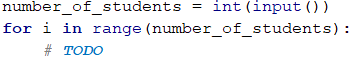Before executing the code of the for loop, we will create variables where we will store the number of students for each group: poor results (up to 2.99), results from 3 to 3.99, from 4 to 4.99, and grades above 5. We will also need one more variable, where we will store the sum of all grades, via which we will calculate the average grade of all students: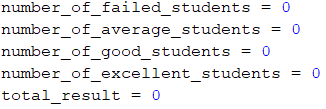We run the loop and inside it, we declare one more variable, in which we will store the currently entered grade. The variable will be of the type float and on every iteration, we check what is its value. According to this value, we increase the number of students in the relevant group by 1, as we should not forget to also increase the total amount of the grades, which we also track: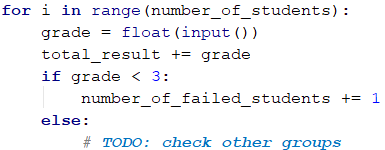We can calculate what percentage is taken by a particular group of students from the total number by multiplying the number of students in the relevant group by 100 and then dividing this by the total number of students.

The final result is formed in a well-known way up to the second symbol after the decimal point.

## Problem: Christmas Hat

Write a program that reads from the console an integer n and draws a Christmas hat with a width of 4 * n + 1 columns and a height of 2 * n + 5 rows, as in the examples below.

### Input Data

The input is read from the console - one integer n within the range [3 … 100].

### Output Data

Print on the console a Christmas hat, exactly like in the examples.

### Sample Input and Output

Input Output
4 ......./|\.......
.......\|/.......
.......***.......
......*-*-*......
.....*--*--*.....
....*---*---*....
...*----*----*...
..*-----*-----*..
.*------*------*.
*-------*-------*
*****************
*.*.*.*.*.*.*.*.*
*****************
Input Output
7 ............./|\.............
.............\|/.............
.............***.............
............*-*-*............
...........*--*--*...........
..........*---*---*..........
.........*----*----*.........
........*-----*-----*........
.......*------*------*.......
......*-------*-------*......
.....*--------*--------*.....
....*---------*---------*....
...*----------*----------*...
..*-----------*-----------*..
.*------------*------------*.
*-------------*-------------*
*****************************
*.*.*.*.*.*.*.*.*.*.*.*.*.*.*
*****************************

### Hints and Guidelines

In tasks requiring drawing on the console, most often the user inputs an integer that is related to the total size of the figure that we need to draw. As the task requirements mention how the total length and width of the figure are calculated, we can use them as starting points. In the examples, it is clear that regardless of the input data, we always have the first two rows that are almost identical.

......./|\.......
.......\|/.......

We also notice that the last three rows are always present, as two of them are completely the same.

*****************
*.*.*.*.*.*.*.*.*
*****************

By these observations, we can come up with the formula for the height of the variable part of the Christmas hat. We use the formula specified in the task to calculate the total height, by subtracting the size of the unchangeable part. We obtain (2 * n + 5) – 5 or 2 * n.

To draw the dynamic or the variable part of the figure, we will use a loop. The size of the loop will be from 0 to the width that we have by requirements, namely 4 * n + 1. Since we will use this formula in a few places in the code, it is a good practice to declare it in a separate variable. Before running the loop, we should declare variables for the number of individual symbols that participate in the dynamic part: dots and dashes. By analyzing examples, we can also prepare formulas for the starting values of these variables. Initially, the dashes are 0, but we can calculate the number of dots by subtracting 3 from the total width (the number of symbols that are building the top of the Christmas hat) and then dividing by 2, as the number of dots on both sides of the hat is the same.

.......***.......
......*-*-*......
.....*--*--*.....
....*---*---*....
...*----*----*...
..*-----*-----*..
.*------*------*.
*-------*-------*

What remains is to execute the body of the loop, as after each drawing we decrease the number of dots by 1 and increase the number of dashes by 1. Let's not forget to draw one star between each of them. The sequence of drawing in the body of the loop is the following:

• Symbol string of dots
• Star
• Symbol string of dashes
• Star
• Symbol string of dashes
• Star
• Symbol string of dots

In case we have worked properly, we will obtain figures identical to those in the examples.

## Problem: Letters Combinations

Write a program that prints on the console all combinations of 3 letters within a specified range, by skipping the combinations containing a certain letter. Finally, print the number of printed combinations.

### Input Data

The input is read from the console and contains exactly 3 lines:

• A small letter from the English alphabet – between 'a' and 'z'.
• A small letter from the English alphabet – between the first letter and 'z'.
• A small letter from the English alphabet – from 'a' to 'z' – as the combinations containing this letter are skipped.

### Output Data

Print on one line all combinations corresponding to the requirements, followed by their number, separated by a space.

### Sample Input and Output

a
c
b
aaa aac aca acc caa cac cca ccc 8 All possible combinations with letters 'a', 'b' and 'c' are:
aaa aab aac aba abb abc aca acb acc baa bab bac bba bbb bbc bca bcb bcc caa cab cac cba cbb cbc cca ccb ccc
The combinations containing 'b' are not valid.
8 valid combinations remain.
Input Output
f
k
h
fff ffg ffi ffj ffk fgf fgg fgi fgj fgk fif fig fii fij fik fjf fjg fji fjj fjk fkf fkg fki fkj fkk gff gfg gfi gfj gfk ggf ggg ggi ggj ggk gif gig gii gij gik gjf gjg gji gjj gjk gkf gkg gki gkj gkk iff ifg ifi ifj ifk igf igg igi igj igk iif iig iii iij iik ijf ijg iji ijj ijk ikf ikg iki ikj ikk jff jfg jfi jfj jfk jgf jgg jgi jgj jgk jif jig jii jij jik jjf jjg jji jjj jjk jkf jkg jki jkj jkk kff kfg kfi kfj kfk kgf kgg kgi kgj kgk kif kig kii kij kik kjf kjg kji kjj kjk kkf kkg kki kkj kkk 125
Input Output
a
c
z
aaa aab aac aba abb abc aca acb acc baa bab bac bba bbb bbc bca bcb bcc caa cab cac cba cbb cbc cca ccb ccc 27

### Hints and Guidelines

By requirements, we have input data on 3 rows, each of which is represented by one character of the ASCII table (https://www.asciitable.com/). We could use the already defined function in Python, ord(…) through which we will receive the ASCII code of the symbol: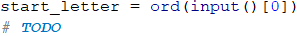Let's think of how we can achieve the final result. In case the task requirement is to print all characters, from the starting to the end one (by skipping a particular letter), what should we do?

The easiest and most efficient way is to use a loop, by passing through all characters and printing those that are different from the letter that we need to skip. In Python, we can go around all the symbols from 'a' to 'z' the following way: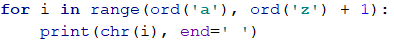The function chr(…) will convert the received ASCII code into a symbol. The result of running the code is all letters from a to z included, printed on a single line and separated by spaces. Does this look like the final result of our task? We must find a way to print 3 characters, as required, instead of 1. Running such a program very much looks like a slot machine. We often win in slots, if we arrange a few identical characters in a row. Let's say that the machine has space for three characters. When we stop on a particular character in the first place, the other two places will continue rolling characters among all possible ones. In our case, all possible characters are the letters from the starting to the end one, entered by the user, and the solution of our program is identical to the way a slot machine works.

We use a loop that runs through all characters from the starting to the end letter (included). On each iteration of the first loop, we run a second one with the same parameters (but only if the letter of the first loop is valid, i.e. does not match the one that we must exclude, by requirements). In each iteration of the second loop, we run one more with the same parameters and the same condition. This way we have three nested loops and in the body of the latter we will add the symbols to the final result: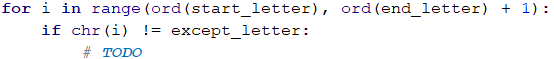Let's not forget that we also need to print the total number of valid combinations that we have found, and they must be printed on the same line, separated by a space. We leave this sub-task to the reader.